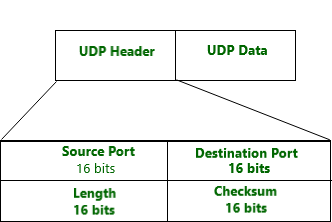User Datagram Protocol is a Transport Layer protocol. UDP is a part of the Internet Protocol suite, referred to as UDP/IP suite. Unlike TCP, it is an unreliable and connectionless protocol. So, there is no need to establish a connection prior to data transfer. Though Transmission Control Protocol (TCP) is the dominant transport layer protocol used with most Internet services; provides assured delivery, reliability, and much more but all these services cost us additional overhead and latency. Here, UDP comes into the picture. For real-time services like computer gaming, voice or video communication, live conferences; we need UDP. Since high performance is needed, UDP permits packets to be dropped instead of processing delayed packets. There is no error checking in UDP, so it also saves bandwidth.
User Datagram Protocol (UDP) is more efficient in terms of both latency and bandwidth.UDP header is an 8-bytes fixed and simple header. The first 8 Bytes contains all necessary header information and the remaining part consist of data. UDP port number fields are each 16 bits long, therefore the range for port numbers defined from 0 to 65535; port number 0 is reserved. Port numbers help to distinguish different user requests or processes.Example-1 :
Given a DUMP of a UDP header in hexadecimal format 06 32 00 0D 00 1C E2 17. Find the following:-

1. Source port number?
2. Destination port number?
3. Length of user datagram?
4. Length of the data?

Solution :

1. Source Port –
Source Port is 2 Byte long field used to identify the port number of the source. The source port number is the first four hexadecimal digits i.e. 06 32 if we convert hexadecimal to decimal we get 1586.
2. Destination Port –
It is a 2 Byte long field, used to identify the port of the destined packet. The destination port number is the second four hexadecimal digits 00 0D if we convert hexadecimal to decimal we get 13
3. Length –
Length is the length of UDP including the header and the data. It is a 16-bits field. The third four hexadecimal digits 00 1C if we convert hexadecimal to decimal we get 16 define the length of the whole UDP packet as 28
4. Length of the header –
It is 8 bytes as it is fixed. The length of the data is the length of the whole packet — the length of the header i.e. 28 – 8 = 20 bytes.

Example-2 :

Given a DUMP of a UDP header in hexadecimal format 04 21 00 0B 00 2A E2 17. Find the following:-

1. Source port number?
2. Destination port number?
3. Length of user datagram?
4. Length of the data?

Solution:

1. The source port number is the first four hexadecimal digits i.e. 04 21 if we convert hexadecimal to decimal we get 1057
2. The destination port number is the second four hexadecimal digits 00 0B if we convert hexadecimal to decimal we get 11
3. The third four hexadecimal digits 00 2A if we convert hexadecimal to decimal 42 define the length of the whole UDP packet as 28
4. The length of the data is the length of the whole packet — the length of the header i.e. 28 – 8 = 20 bytes.

Example-3 :

Given a DUMP of a UDP header in hexadecimal format 03 61 10 1A 10 4C Y2 42. Find the following:-

1. Source port number?
2. Destination port number?
3. Length of user datagram?
4. Length of the data?

Solution:

1. The source port number is the first four hexadecimal digits i.e. 03 61 if we convert hexadecimal to decimal we get 0865
2. The destination port number is the second four hexadecimal digits 10 1A if we convert hexadecimal to decimal we get 4122
3. The third four hexadecimal digits 10 4C if we convert hexadecimal to decimal 4172 define the length of the whole UDP packet as 28
4. The length of the data is the length of the whole packet — the length of the header i.e. 28 – 8 = 20 bytes.

Note :
UDP header also contains payload data which is of variable length. Use of UDP is as a tunneling protocol, where a tunnel endpoint encapsulates the packets of another protocol inside UDP datagrams and transmits them to another tunnel endpoint, which decapsulates the UDP datagrams and forwards the original packets contained in the payload.